# Tag: representations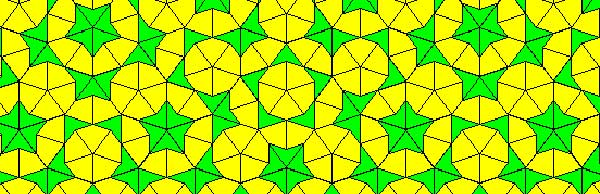Penrose tilings are aperiodic tilings of the plane, made from 2 sort of tiles : kites and darts. It is well known (see for example the standard textbook tilings and patterns section 10.5) that one can describe a Penrose tiling around a given point in the plane as an infinite sequence of 0’s and 1’s, subject to the condition that no two consecutive 1’s appear in the sequence. Conversely, any such sequence is the sequence of a Penrose tiling together with a point. Moreover, if two such sequences are eventually the same (that is, they only differ in the first so many terms) then these sequences belong to two points in the same tiling,

Another remarkable feature of Penrose tilings is their local isomorphism : fix a finite region around a point in one tiling, then in any other Penrose tiling one can find a point having an isomorphic region around it. For this reason, the space of all Penrose tilings has horrible topological properties (all points lie in each others closure) and is therefore a prime test-example for the techniques of noncommutative geometry.

In his old testament, Noncommutative Geometry, Alain Connes associates to this space a $C^*$-algebra $Fib$ (because it is constructed from the Fibonacci series $F_0,F_1,F_2,…$) which is the direct limit of sums of two full matrix-algebras $S_n$, with connecting morphisms

$S_n = M_{F_n}(\mathbb{C}) \oplus M_{F_{n-1}}(\mathbb{C}) \rightarrow S_{n+1} = M_{F_{n+1}}(\mathbb{C}) \oplus M_{F_n}(\mathbb{C}) \qquad (a,b) \mapsto ( \begin{matrix} a & 0 \\ 0 & b \end{matrix}, a)$

As such $Fib$ is an AF-algebra (for approximately finite) and hence formally smooth. That is, $Fib$ would be the coordinate ring of a smooth variety in the noncommutative sense, if only $Fib$ were finitely generated. However, $Fib$ is far from finitely generated and has other undesirable properties (at least for a noncommutative algebraic geometer) such as being simple and hence in particular $Fib$ has no finite dimensional representations…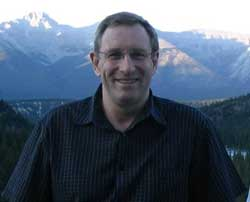A couple of weeks ago, Paul Smith discovered a surprising connection between the noncommutative space of Penrose tilings and an affine algebra in the paper The space of Penrose tilings and the non-commutative curve with homogeneous coordinate ring $\mathbb{C} \langle x,y \rangle/(y^2)$.

Giving $x$ and $y$ degree 1, the algebra $P = \mathbb{C} \langle x,y \rangle/(y^2)$ is obviously graded and noncommutative projective algebraic geometers like to associate to such algebras their ‘proj’ which is the quotient category of the category of all graded modules in which two objects become isomorphisc iff their ‘tails’ (that is forgetting the first few homogeneous components) are isomorphic.

The first type of objects NAGers try to describe are the point modules, which correspond to graded modules in which every homogeneous component is 1-dimensional, that is, they are of the form

$\mathbb{C} e_0 \oplus \mathbb{C} e_1 \oplus \mathbb{C} e_2 \oplus \cdots \oplus \mathbb{C} e_n \oplus \mathbb{C} e_{n+1} \oplus \cdots$

with $e_i$ an element of degree $i$. The reason for this is that point-modules correspond to the points of the (usual, commutative) projective variety when the affine graded algebra is commutative.

Now, assume that a Penrose tiling has been given by a sequence of 0’s and 1’s, say $(z_0,z_1,z_2,\cdots)$, then it is easy to associate to it a graded vectorspace with action given by

$x.e_i = e_{i+1}$ and $y.e_i = z_i e_{i+1}$

Because the sequence has no two consecutive ones, it is clear that this defines a graded module for the algebra $P$ and determines a point module in $\pmb{proj}(P)$. By the equivalence relation on Penrose sequences and the tails-equivalence on graded modules it follows that two sequences define the same Penrose tiling if and only if they determine the same point module in $\pmb{proj}(P)$. Phrased differently, the noncommutative space of Penrose tilings embeds in $\pmb{proj}(P)$ as a subset of the point-modules for $P$.

The only such point-module invariant under the shift-functor is the one corresponding to the 0-sequence, that is, corresponds to the cartwheel tiling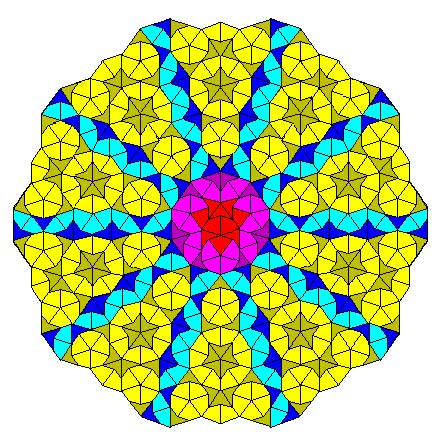Another nice consequence is that we can now explain the local isomorphism property of Penrose tilings geometrically as a consequence of the fact that the $Ext^1$ between any two such point-modules is non-zero, that is, these noncommutative points lie ‘infinitely close’ to each other.

This is the easy part of Paul’s paper.

The truly, truly amazing part is that he is able to recover Connes’ AF-algebra $Fib$ from $\pmb{proj}(P)$ as the algebra of global sections! More precisely, he proves that there is an equivalence of categories between $\pmb{proj}(P)$ and the category of all $Fib$-modules $\pmb{mod}(Fib)$!

In other words, the noncommutative projective scheme $\pmb{proj}(P)$ is actually isomorphic to an affine scheme and as its coordinate ring is formally smooth $\pmb{proj}(P)$ is a noncommutative smooth variety. It would be interesting to construct more such examples of interesting AF-algebras appearing as local rings of sections of proj-es of affine graded algebras.

A few years ago a student entered my office asking suggestions for his master thesis.

“I’m open to any topic as long as it has nothing to do with those silly quivers!”

At that time not the best of opening-lines to address me and, inevitably, the most disastrous teacher-student-conversation-ever followed (also on my part, i’m sorry to say).

This week, Markus Reineke had a similar, though less confrontational, experience. Markus gave a mini-course on ‘moduli spaces of representations’ in our advanced master class. Students loved the way he introduced representation varieties and constructed the space of irreducible representations as a GIT-quotient. In fact, his course was probably the first in that program having an increasing (rather than decreasing) number of students attending throughout the week…

In his third lecture he wanted to illustrate these general constructions and what better concrete example to take than representations of quivers? Result : students’ eyes staring blankly at infinity…

What is it that quivers do to have this effect on students?

Perhaps quiver-representations cause them an information-overload.

Perhaps we should take plenty of time to explain that in going from the quiver (the directed graph) to the path algebra, vertices become idempotents and arrows the remaining generators. These idempotents split a representation space into smaller vertex-spaces, the dimensions of which we collect in a dimension-vector, the big basechange group splits therefore into a product of small vertex-basechanges and the action of this product on an matrix corresponding to an arrow is merely usual conjugation by the big basechange-group, etc. etc. Blatant trivialities to someone breathing quivers, but probably we too had to take plenty of time once to disentangle this information-package…

But then, perhaps they consider quivers and their representations as too-concrete-old-math-stuff, when there’s so much high-profile-fancy-math still left to taste.

When given the option, students prefer you to tell them monstrous-moonshine stories even though they can barely prove simplicity of $A_5$, they want you to give them a short-cut to the Langlands programme but have never had the patience nor the interest to investigate the splitting of primes in quadratic number fields, they want to be taught schemes and their structure sheaves when they still struggle with the notion of a dominant map between varieties…

In short, students often like to run before they can crawl.

Working through the classification of some simple quiver-settings would force their agile feet firmly on the ground. They probably experience this as a waste of time.

Perhaps, it is time to promote slow math…

The Knight-seating problems asks for a consistent placing of n-th Knight at an odd root of unity, compatible with the two different realizations of the algebraic closure of the field with two elements.
The first identifies the multiplicative group of its non-zero elements with the group of all odd complex roots of unity, under complex multiplication. The second uses Conway’s ‘simplicity rules’ to define an addition and multiplication on the set of all ordinal numbers.

The odd Knights of the round table-problem asks for a specific one-to-one correspondence between two realizations of ‘the’ algebraic closure $\overline{\mathbb{F}_2}$ of the field of two elements.

The first identifies the multiplicative group of its non-zero elements with the group of all odd complex roots of unity, under complex multiplication. The addition on $\overline{\mathbb{F}_2}$ is then recovered by inducing an involution on the odd roots, pairing the one corresponding to x to the one corresponding to x+1.

The second uses Conway’s ‘simplicity rules’ to define an addition and multiplication on the set of all ordinal numbers. Conway proves in ONAG that this becomes an algebraically closed field of characteristic two and that $\overline{\mathbb{F}_2}$ is the subfield of all ordinals smaller than $\omega^{\omega^{\omega}}$. The finite ordinals (the natural numbers) form the quadratic closure of $\mathbb{F}_2$.

On the natural numbers the Conway-addition is binary addition without carrying and Conway-multiplication is defined by the properties that two different Fermat-powers $N=2^{2^i}$ multiply as they do in the natural numbers, and, Fermat-powers square to its sesquimultiple, that is $N^2=\frac{3}{2}N$. Moreover, all natural numbers smaller than $N=2^{2^{i}}$ form a finite field $\mathbb{F}_{2^{2^i}}$. Using distributivity, one can write down a multiplication table for all 2-powers.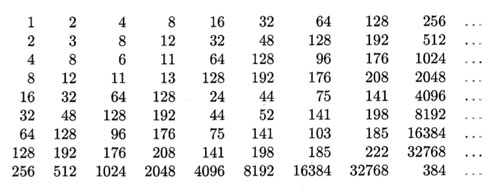The Knight-seating problems asks for a consistent placing of n-th Knight $K_n$ at an odd root of unity, compatible with the two different realizations of $\overline{\mathbb{F}_2}$. Last time, we were able to place the first 15 Knights as below, and asked where you would seat $K_{16}$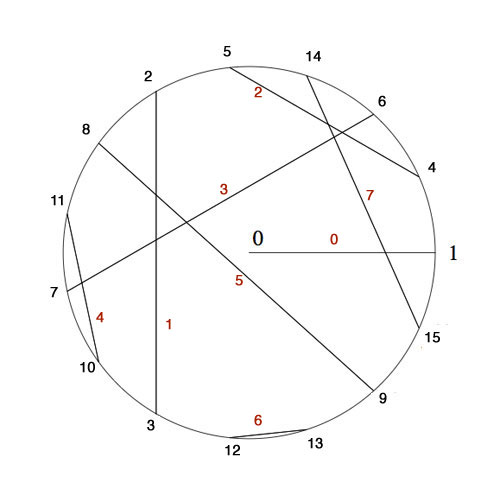$K_4$ was placed at $e^{2\pi i/15}$ as 4 was the smallest number generating the ‘Fermat’-field $\mathbb{F}_{2^{2^2}}$ (with multiplicative group of order 15) subject to the compatibility relation with the generator 2 of the smaller Fermat-field $\mathbb{F}_2$ (with group of order 15) that $4^5=2$.

To include the next Fermat-field $\mathbb{F}_{2^{2^3}}$ (with multiplicative group of order 255) consistently, we need to find the smallest number n generating the multiplicative group and satisfying the compatibility condition $n^{17}=4$. Let’s first concentrate on finding the smallest generator : as 2 is a generator for 1st Fermat-field $\mathbb{F}_{2^{2^1}}$ and 4 a generator for the 2-nd Fermat-field $\mathbb{F}_{2^{2^2}}$ a natural conjecture might be that 16 is a generator for the 3-rd Fermat-field $\mathbb{F}_{2^{2^3}}$ and, more generally, that $2^{2^i}$ would be a generator for the next field $\mathbb{F}_{2^{2^{i+1}}}$.

However, an “exercise” in the 1978-paper by Hendrik Lenstra Nim multiplication asks : “Prove that $2^{2^i}$ is a primitive root in the field $\mathbb{F}_{2^{2^{i+1}}}$ if and only if i=0 or 1.”

I’ve struggled with several of the ‘exercises’ in Lenstra’s paper to the extend I feared Alzheimer was setting in, only to find out, after taking pen and paper and spending a considerable amount of time calculating, that they are indeed merely exercises, when looked at properly… (Spoiler-warning : stop reading now if you want to go through this exercise yourself).

In the picture above I’ve added in red the number $x(x+1)=x^2+1$ to each of the involutions. Clearly, for each pair these numbers are all distinct and we see that for the indicated pairing they make up all numbers strictly less than 8.

By Conway’s simplicity rules (or by checking) the pair (16,17) gives the number 8. In other words, the equation
$x^2+x+8$ is an irreducible polynomial over $\mathbb{F}_{16}$ having as its roots in $\mathbb{F}_{256}$ the numbers 16 and 17. But then, 16 and 17 are conjugated under the Galois-involution (the Frobenius $y \mapsto y^{16}$). That is, we have $16^{16}=17$ and $17^{16}=16$ and hence $16^{17}=8$. Now, use the multiplication table in $\mathbb{F}_{16}$ given in the previous post (or compute!) to see that 8 is of order 5 (and NOT a generator). As a consequence, the multiplicative order of 16 is 5×17=85 and so 16 cannot be a generator in $\mathbb{F}_{256}$.
For general i one uses the fact that $2^{2^i}$ and $2^{2^i}+1$ are the roots of the polynomial $x^2+x+\prod_{j<i} 2^{2^j}$ over $\mathbb{F}_{2^{2^i}}$ and argues as before.

Right, but then what is the minimal generator satisfying $n^{17}=4$? By computing we see that the pairings of all numbers in the range 16…31 give us all numbers in the range 8…15 and by the above argument this implies that the 17-th powers of all numbers smaller than 32 must be different from 4. But then, the smallest candidate is 32 and one verifies that indeed $32^{17}=4$ (use the multiplication table given before).

Hence, we must place Knight $K_{32}$ at root $e^{2 \pi i/255}$ and place the other Knights prior to the 256-th at the corresponding power of 32. I forgot the argument I used to find-by-hand the requested place for Knight 16, but one can verify that $32^{171}=16$ so we seat $K_{16}$ at root $e^{342 \pi i/255}$.

But what about Knight $K_{256}$? Well, by this time I was quite good at squaring and binary representations of integers, but also rather tired, and decided to leave that task to the computer.

If we denote Nim-addition and multiplication by $\oplus$ and $\otimes$, then Conway’s simplicity results in ONAG establish a field-isomorphism between $~(\mathbb{N},\oplus,\otimes)$ and the field $\mathbb{F}_2(x_0,x_1,x_2,\ldots )$ where the $x_i$ satisfy the Artin-Schreier equations

$x_i^2+x_i+\prod_{j < i} x_j = 0$

and the i-th Fermat-field $\mathbb{F}_{2^{2^i}}$ corresponds to $\mathbb{F}_2(x_0,x_1,\ldots,x_{i-1})$. The correspondence between numbers and elements from these fields is given by taking $x_i \mapsto 2^{2^i}$. But then, wecan write every 2-power as a product of the $x_i$ and use the binary representation of numbers to perform all Nim-calculations with numbers in these fields.

Therefore, a quick and dirty way (and by no means the most efficient) to do Nim-calculations in the next Fermat-field consisting of all numbers smaller than 65536, is to use sage and set up the field $\mathbb{F}_2(x_0,x_1,x_2,x_3)$ by

R.< x,y,z,t > =GF(2)[]
S.< a,b,c,d >=R.quotient((x^2+x+1,y^2+y+x,z^2+z+x*y,t^2+t+x*y*z))


To find the smallest number generating the multiplicative group and satisfying the additional compatibility condition $n^{257}=32$ we have to find the smallest binary number $i_1i_2 \ldots i_{16}$ (larger than 255) satisfying

(i1*a*b*c*t+i2*b*c*t+i3*a*c*t+i4*c*t+i5*a*b*t+i6*b*t+
i7*a*t+i8*t+i9*a*b*c+i10*b*c+i11*a*c+i12*c+i13*a*b+
i14*b+i15*a+i16)^257=a*c


It takes a 2.4GHz 2Gb-RAM MacBook not that long to decide that the requested generator is 1051 (killing another optimistic conjecture that these generators might be 2-powers). So, we seat Knight
$K_{1051}$ at root $e^{2 \pi i/65535}$ and can then arrange seatings for all Knight queued up until we reach the 65536-th! In particular, the first Knight we couldn’t place before, that is Knight $K_{256}$, will be seated at root $e^{65826 \pi i/65535}$.

If you’re lucky enough to own a computer with more RAM, or have the patience to make the search more efficient and get the seating arrangement for the next Fermat-field, please drop a comment.

I’ll leave you with another Lenstra-exercise which shouldn’t be too difficult for you to solve now : “Prove that $x^3=2^{2^i}$ has three solutions in $\mathbb{N}$ for each $i \geq 2$.”

I really like Matilde Marcolli’s idea to use some of Jackson Pollock’s paintings as metaphors for noncommutative spaces. In her talk she used this painting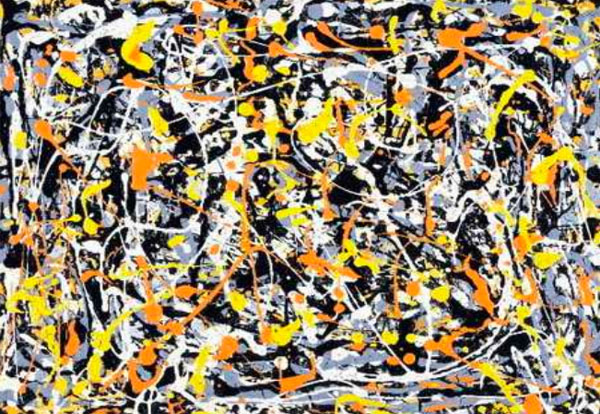and refered to it (as did I in my post) as : Jackson Pollock “Untitled N.3”. Before someone writes a post ‘The Pollock noncommutative space hoax’ (similar to my own post) let me point out that I am well aware of the controversy surrounding this painting.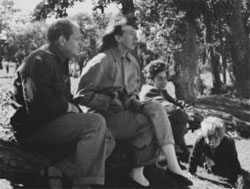This painting is among 32 works recently discovered and initially attributed to Pollock.
In fact, I’ve already told part of the story in Doodles worth millions (or not)? (thanks to PD1). The story involves the people on the right : from left to right, Jackson Pollock, his wife Lee Krasner, Mercedes Matter and her son Alex Matter.

Alex Matter, whose father, Herbert, and mother, Mercedes, were artists and friends of Jackson Pollock, discovered after his mother died a group of small drip paintings in a storage locker in Wainscott, N.Y. which he believed to be authentic Pollocks.

Read the post mentioned above if you want to know how mathematics screwed up his plan, or much better, reed the article Anatomy of the Jackson Pollock controversy by Stephen Litt.

So, perhaps the painting above was not the smartest choice, but we could take any other genuine Pollock ‘drip-painting’, a technique he taught himself towards the end of 1946 to make an image by splashing, pouring, sloshing colors onto the canvas. Typically, such a painting consists of blops of paint, connected via thin drip-lines.

What does this have to do with noncommutative geometry? Well, consider the blops as ‘points’. In commutative geometry, distinct points cannot share tangent information ((technically : a commutative semi-local ring splits as the direct sum of local rings and this does no longer hold for a noncommutative semi-local ring)). In the noncommutative world though, they can!, or if you want to phrase it like this, noncommutative points ‘can talk to each other’. And, that’s what we cherish in those drip-lines.

But then, if two points share common tangent informations, they must be awfully close to each other… so one might imagine these Pollock-lines to be strings holding these points together. Hence, it would make more sense to consider the ‘Pollock-quotient-painting’, that is, the space one gets after dividing out the relation ‘connected by drip-lines’ ((my guess is that Matilde thinks of the lines as the action of a group on the points giving a topological horrible quotient space, and thats precisely where noncommutative geometry shines)).

For this reason, my own mental picture of a genuinely noncommutative space ((that is, the variety corresponding to a huge noncommutative algebra such as free algebras, group algebras of arithmetic groups or fundamental groups)) looks more like the picture below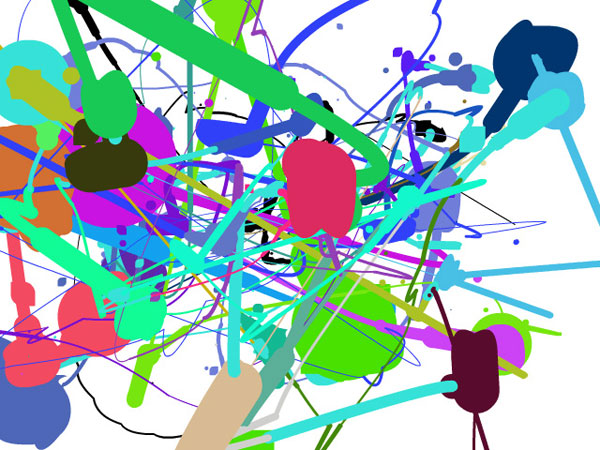The colored blops you see are really sets of points which you might view as, say, a FacebookGroup ((technically, think of them as the connected components of isomorphism classes of finite dimensional simple representations of your favorite noncommutative algebra)). Some chatter may occur between two distinct FacebookGroups, the more chatter the thicker the connection depicted ((technically, the size of the connection is the dimension of the ext-group between generic simples in the components)). Now, there are some tiny isolated spots (say blue ones in the upper right-hand quadrant). These should really be looked at as remote clusters of noncommutative points (sharing no (tangent) information whatsoever with the blops in the foregound). If we would zoom into them beyond the Planck scale (if I’m allowed to say a bollock-word in a Pollock-post) they might reveal again a whole universe similar to the interconnected blops upfront.

The picture was produced using the fabulous Pollock engine. Just use your mouse to draw and click to change colors in order to produce your very own noncommutative space!

For the mathematicians still around, this may sound like a lot of Pollock-bollocks but can be made precise. See my note Noncommutative geometry and dual coalgebras for a very terse reading. Now that coalgebras are gaining popularity, I really should write a more readable account of it, including some fanshi-wanshi examples…

Conway and Norton showed that there are exactly 171 moonshine functions and associated two arithmetic subgroups to them. We want a tool to describe these and here’s where Conway’s big picture comes in very handy. All moonshine groups are arithmetic groups, that is, they are commensurable with the modular group. Conway’s idea is to view several of these groups as point- or set-wise stabilizer subgroups of finite sets of (projective) commensurable 2-dimensional lattices.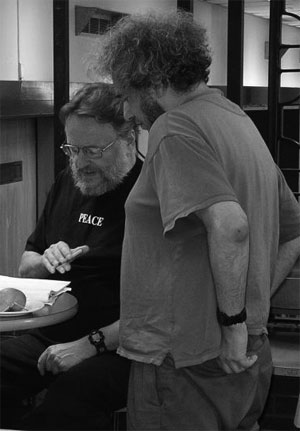Expanding (and partially explaining) the original moonshine observation of McKay and Thompson, John Conway and Simon Norton formulated monstrous moonshine :

To every cyclic subgroup $\langle m \rangle$ of the Monster $\mathbb{M}$ is associated a function

$f_m(\tau)=\frac{1}{q}+a_1q+a_2q^2+\ldots$ with $q=e^{2 \pi i \tau}$ and all coefficients $a_i \in \mathbb{Z}$ are characters at $m$ of a representation of $\mathbb{M}$. These representations are the homogeneous components of the so called Moonshine module.

Each $f_m$ is a principal modulus for a certain genus zero congruence group commensurable with the modular group $\Gamma = PSL_2(\mathbb{Z})$. These groups are called the moonshine groups.

Conway and Norton showed that there are exactly 171 different functions $f_m$ and associated two arithmetic subgroups $F(m) \subset E(m) \subset PSL_2(\mathbb{R})$ to them (in most cases, but not all, these two groups coincide).

Whereas there is an extensive literature on subgroups of the modular group (see for instance the series of posts starting here), most moonshine groups are not contained in the modular group. So, we need a tool to describe them and here’s where Conway’s big picture comes in very handy.

All moonshine groups are arithmetic groups, that is, they are subgroups $G$ of $PSL_2(\mathbb{R})$ which are commensurable with the modular group $\Gamma = PSL_2(\mathbb{Z})$ meaning that the intersection $G \cap \Gamma$ is of finite index in both $G$ and in $\Gamma$. Conway’s idea is to view several of these groups as point- or set-wise stabilizer subgroups of finite sets of (projective) commensurable 2-dimensional lattices.

Start with a fixed two dimensional lattice $L_1 = \mathbb{Z} e_1 + \mathbb{Z} e_2 = \langle e_1,e_2 \rangle$ and we want to name all lattices of the form $L = \langle v_1= a e_1+ b e_2, v_2 = c e_1 + d e_2 \rangle$ that are commensurable to $L_1$. Again this means that the intersection $L \cap L_1$ is of finite index in both lattices. From this it follows immediately that all coefficients $a,b,c,d$ are rational numbers.

It simplifies matters enormously if we do not look at lattices individually but rather at projective equivalence classes, that is $~L=\langle v_1, v_2 \rangle \sim L’ = \langle v’_1,v’_2 \rangle$ if there is a rational number $\lambda \in \mathbb{Q}$ such that $~\lambda v_1 = v’_1, \lambda v_2=v’_2$. Further, we are of course allowed to choose a different ‘basis’ for our lattices, that is, $~L = \langle v_1,v_2 \rangle = \langle w_1,w_2 \rangle$ whenever $~(w_1,w_2) = (v_1,v_2).\gamma$ for some $\gamma \in PSL_2(\mathbb{Z})$.
Using both operations we can get any lattice in a specific form. For example,

$\langle \frac{1}{2}e_1+3e_2,e_1-\frac{1}{3}e_2 \overset{(1)}{=} \langle 3 e_1+18e_2,6e_1-2e_2 \rangle \overset{(2)}{=} \langle 3 e_1+18 e_2,38 e_2 \rangle \overset{(3)}{=} \langle \frac{3}{38}e_1+\frac{9}{19}e_2,e_2 \rangle$

Here, identities (1) and (3) follow from projective equivalence and identity (2) from a base-change. In general, any lattice $L$ commensurable to the standard lattice $L_1$ can be rewritten uniquely as $L = \langle Me_1 + \frac{g}{h} e_2,e_2 \rangle$ where $M$ a positive rational number and with $0 \leq \frac{g}{h} < 1$.

Another major feature is that one can define a symmetric hyper-distance between (equivalence classes of) such lattices. Take $L=\langle Me_1 + \frac{g}{h} e_2,e_2 \rangle$ and $L’=\langle N e_1 + \frac{i}{j} e_2,e_2 \rangle$ and consider the matrix

$D_{LL’} = \begin{bmatrix} M & \frac{g}{h} \\ 0 & 1 \end{bmatrix} \begin{bmatrix} N & \frac{i}{j} \\ 0 & 1 \end{bmatrix}^{-1}$ and let $\alpha$ be the smallest positive rational number such that all entries of the matrix $\alpha.D_{LL’}$ are integers, then

$\delta(L,L’) = det(\alpha.D_{LL’}) \in \mathbb{N}$ defines a symmetric hyperdistance which depends only of the equivalence classes of lattices (hyperdistance because the log of it behaves like an ordinary distance).

Conway’s big picture is the graph obtained by taking as its vertices the equivalence classes of lattices commensurable with $L_1$ and with edges connecting any two lattices separated by a prime number hyperdistance. Here’s part of the 2-picture, that is, only depicting the edges of hyperdistance 2.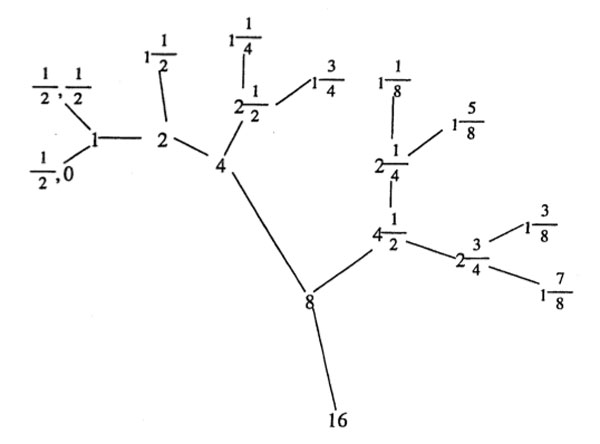The 2-picture is an infinite 3-valent tree as there are precisely 3 classes of lattices at hyperdistance 2 from any lattice $L = \langle v_1,v_2 \rangle$ namely (the equivalence classes of) $\langle \frac{1}{2}v_1,v_2 \rangle~,~\langle v_1, \frac{1}{2} v_2 \rangle$ and $\langle \frac{1}{2}(v_1+v_2),v_2 \rangle$.

Similarly, for any prime hyperdistance p, the p-picture is an infinite p+1-valent tree and the big picture is the product over all these prime trees. That is, two lattices at square-free hyperdistance $N=p_1p_2\ldots p_k$ are two corners of a k-cell in the big picture!
(Astute readers of this blog (if such people exist…) may observe that Conway’s big picture did already appear here prominently, though in disguise. More on this another time).

The big picture presents a simple way to look at arithmetic groups and makes many facts about them visually immediate. For example, the point-stabilizer subgroup of $L_1$ clearly is the modular group $PSL_2(\mathbb{Z})$. The point-stabilizer of any other lattice is a certain conjugate of the modular group inside $PSL_2(\mathbb{R})$. For example, the stabilizer subgroup of the lattice $L_N = \langle Ne_1,e_2 \rangle$ (at hyperdistance N from $L_1$) is the subgroup

${ \begin{bmatrix} a & \frac{b}{N} \\ Nc & d \end{bmatrix}~|~\begin{bmatrix} a & b \\ c & d \end{bmatrix} \in PSL_2(\mathbb{Z})~}$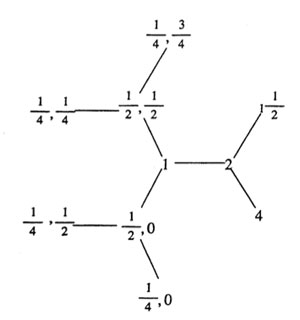Now the intersection of these two groups is the modular subgroup $\Gamma_0(N)$ (consisting of those modular group element whose lower left-hand entry is divisible by N). That is, the proper way to look at this arithmetic group is as the joint stabilizer of the two lattices $L_1,L_N$. The picture makes it trivial to compute the index of this subgroup.

Consider the ball $B(L_1,N)$ with center $L_1$ and hyper-radius N (on the left, the ball with hyper-radius 4). Then, it is easy to show that the modular group acts transitively on the boundary lattices (including the lattice $L_N$), whence the index $[ \Gamma : \Gamma_0(N)]$ is just the number of these boundary lattices. For N=4 the picture shows that there are exactly 6 of them. In general, it follows from our knowledge of all the p-trees the number of all lattices at hyperdistance N from $L_1$ is equal to $N \prod_{p | N}(1+ \frac{1}{p})$, in accordance with the well-known index formula for these modular subgroups!

But, there are many other applications of the big picture giving a simple interpretation for the Hecke operators, an elegant proof of the Atkin-Lehner theorem on the normalizer of $\Gamma_0(N)$ (the whimsical source of appearances of the number 24) and of Helling’s theorem characterizing maximal arithmetical groups inside $PSL_2(\mathbb{C})$ as conjugates of the normalizers of $\Gamma_0(N)$ for square-free N.
J.H. Conway’s paper “Understanding groups like $\Gamma_0(N)$” containing all this material is a must-read! Unfortunately, I do not know of an online version.